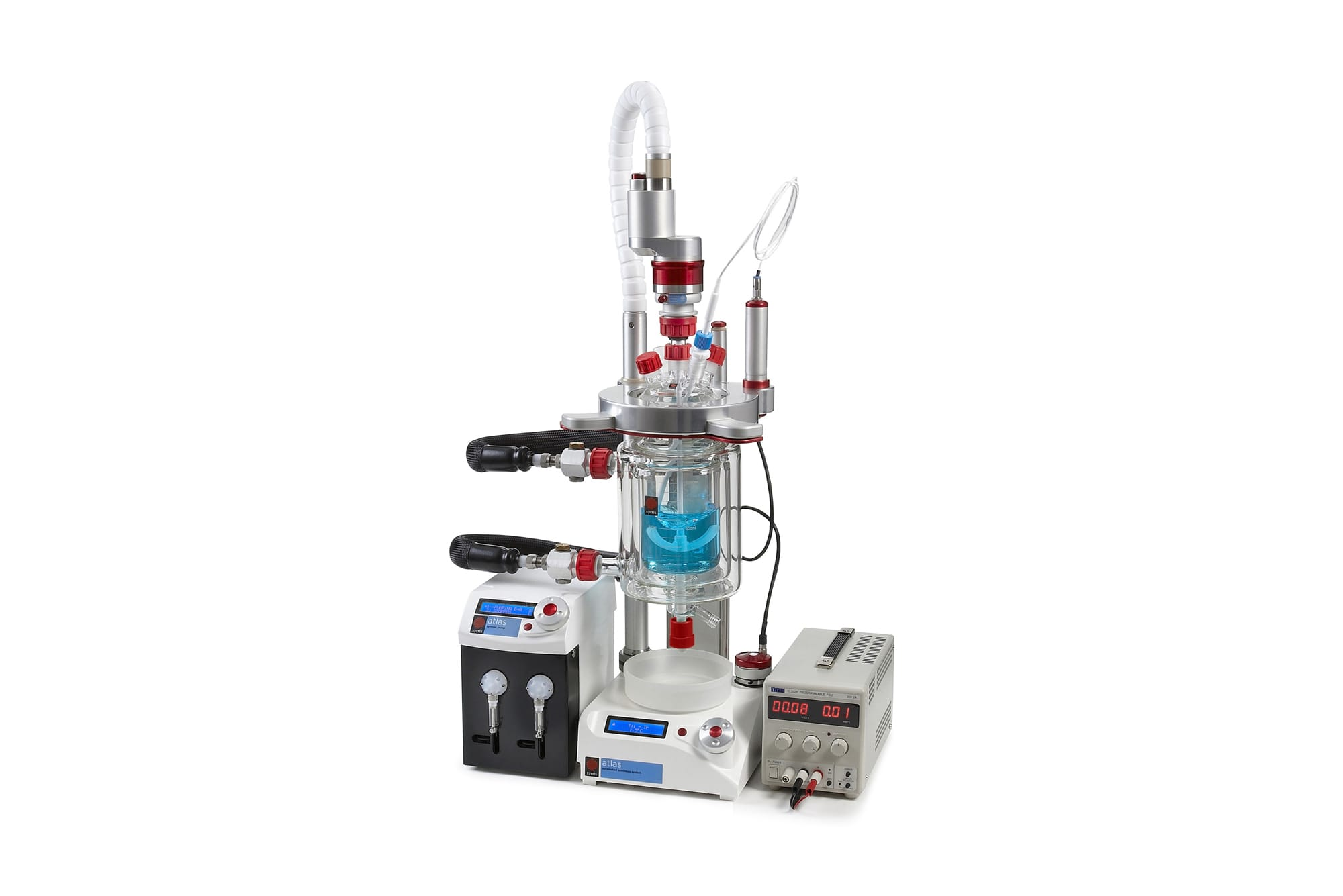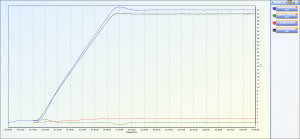## Introduction

The study was performed by a big flavor and perfume manufacturer.

## System configuration

The Atlas HD Calorimeter system was used in its standard configuration (Figure 1).

###Figure 1 – The Atlas HD Calorimeter

## Experiment details

The studied reaction was the hydrolysis of acetic anhydride in presence of a catalytic amount of sulphuric acid.

To an Atlas HD Jacketed Vessel containing 650 mL water and 2g sulphuric acid, 50ml of acetic anhydride was added during 30 min using the Atlas HD Syringe Pump.

The experiment was fully automated using Atlas HD 1.4 Software. The results were analyzed using Atlas HD Reporting Software.

## Hydrolysis of acetic anhydride

### Data processing

CSV file “Ac2O_Water-30min-110613” supplied by Firmenich was processed using Atlas Reporting.

The following experiment details were filled in (Figure 2):

• Initial reactor fill volume: 650 mL
• Reactor fluid: Water
• Added fluid: Acetic anhydride (Note: this fluid type is not available by default and has been added to the fluid list prior to the data analysis)
• Temperature of the added acetic anhydride was assumed to be 25°C

Atlas HD Reporting software automatically generated the two following calorimetry graphs:

• Overall power (Figure 3)
• Enthalpy (Figure 4)The overall power graph (Figure 3) gives us the following data:

• Generated overall power: +17W
• This shows that the reaction is exothermic, as expected

The Enthalpy graph (Figure 4) provides us with the following data:

• Overall Enthalpy: 11kJ
• This is the total enthalpy measured during this experiment
• This is the enthalpy generated by adding a reagent at room temperature to a reaction mixture at a different temperature
• Reaction Enthalpy: 96kJ
• This is the enthalpy of the reaction itself, calculated by deducing the enthalpy of addition from the overall enthalpy. This is the data of interest for the chemist

## Calculations

From the Reaction Enthalpy obtained in Atlas HD Reporting, we can calculate the Heat Reaction.

Acetic Anhydride properties:

• Density: 1.082 g.cm-3
• Molecular Mass: 102.09 g.mol-1

Heat of reaction:

?fH°     =          -Reaction Enthalpy / Moles of acetic anhydride

And we know that:

Moles of acetic anhydride       =          Mass of acetic anhydride / Molecular Mass

=          (Density x Volume) / Molecular Mass

Therefore:

?fH°           =          -(Reaction Enthalpy x Molecular Mass) / (Density x Volume)

=          -(30.96 x 102.09) / (1.082 x 48)

?fH°           =          -60.86kJ.mol-1

=          -562kJ.kg-1

The most recent Heat of Reaction (also called Molar Reaction Enthalpy) for the hydrolysis of acetic anhydride listed by the National Institute of Standards and Technology (NIST) is the following:

?fH°     =          -56.6kJ.mol-1

This information can be obtained on the National Institute of Standards and Technology’s website.

The difference between the Heat of Reaction obtained from the Atlas HD Calorimeter and the theoretical Heat of Reaction is 4.36kJ.mol-1 (7.5%).

To discuss your reaction calorimetry requirements, please speak to the Chemistry team.

Get in touch